# Renormalized stress tensor for transPlanckian cosmology Francisco Diego

• Slides: 23
Download presentationRenormalized stress tensor for trans-Planckian cosmology Francisco Diego Mazzitelli Universidad de Buenos Aires ArgentinaPLAN OF THE TALK • Motivation • Semiclassical Einstein equations and renormalization: usual dispersion relation • Modified dispersion relations: adiabatic renormalization • Examples and related works • Conclusions D. Lopez Nacir, C. Simeone and FDM, PRD 2005MOTIVATIONS • scales of cosmological interest today are sub-planckian at the beginning of inflation potential window to observe Planck-scale physics (Brandenberger, Martin, Starobinsky, Niemeyer, Parentani. . ) • quantum gravity suggests modified dispersion relations for quantum fields at high energies • potential implications: - signatures in the power spectrum of CMB - backreaction on the background spacetime metric Aim of this work: handle divergences in the Semiclassical Einstein Equations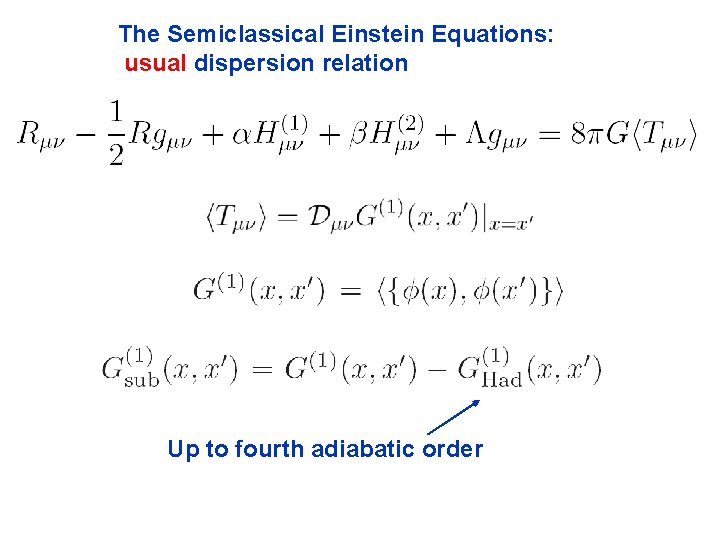The Semiclassical Einstein Equations: usual dispersion relation Up to fourth adiabatic orderThis subtraction works for some quantum states of the scalar field: those for which the two-point function reproduces the Hadamard structure. These are the physical states of theory. The infinities can be absorbed into the gravitational constants in the SEE. Alternative to point-splitting -> dimensional regularizationIn Robertson Walker spacetimes the procedure above is equivalent to the so called adiabatic subtraction: usual dispersion relation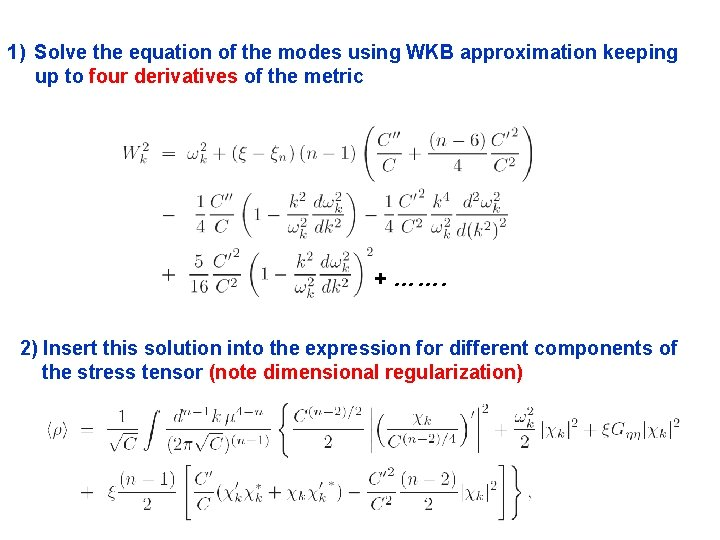1) Solve the equation of the modes using WKB approximation keeping up to four derivatives of the metric + ……. 2) Insert this solution into the expression for different components of the stress tensor (note dimensional regularization)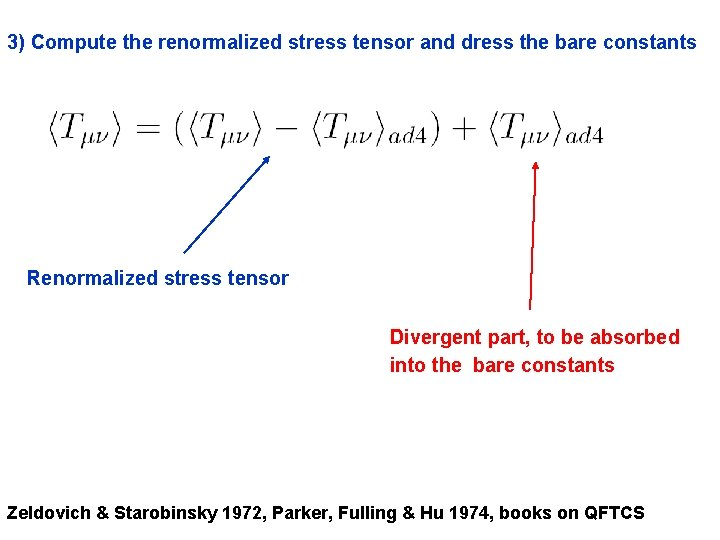3) Compute the renormalized stress tensor and dress the bare constants Renormalized stress tensor Divergent part, to be absorbed into the bare constants Zeldovich & Starobinsky 1972, Parker, Fulling & Hu 1974, books on QFTCSA simpler example: renormalization of Only the zeroth adiabatic order diverges For the numerical evaluation, one can take the n->4 limit inside the integral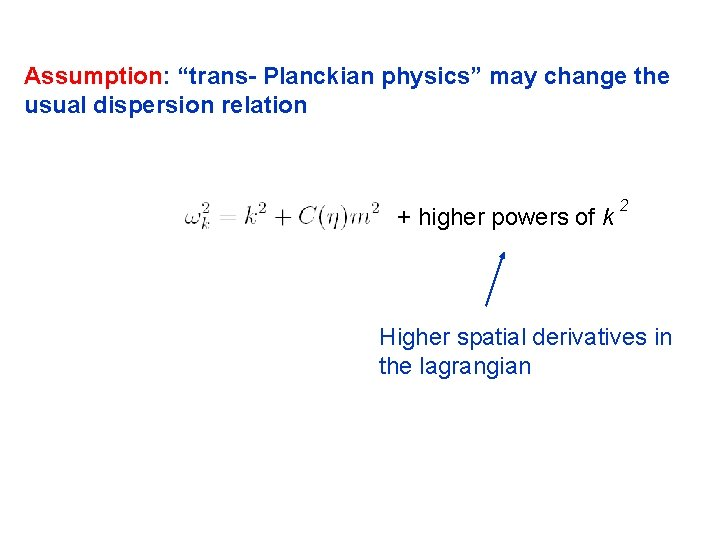Assumption: “trans- Planckian physics” may change the usual dispersion relation + higher powers of k 2 Higher spatial derivatives in the lagrangian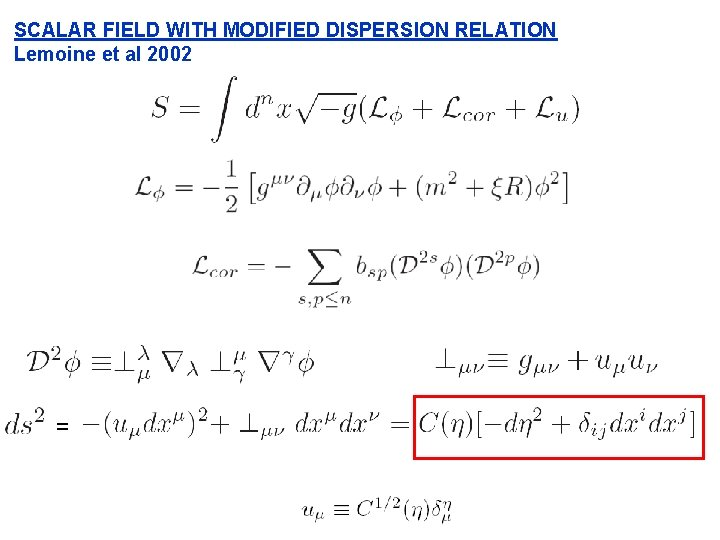SCALAR FIELD WITH MODIFIED DISPERSION RELATION Lemoine et al 2002 =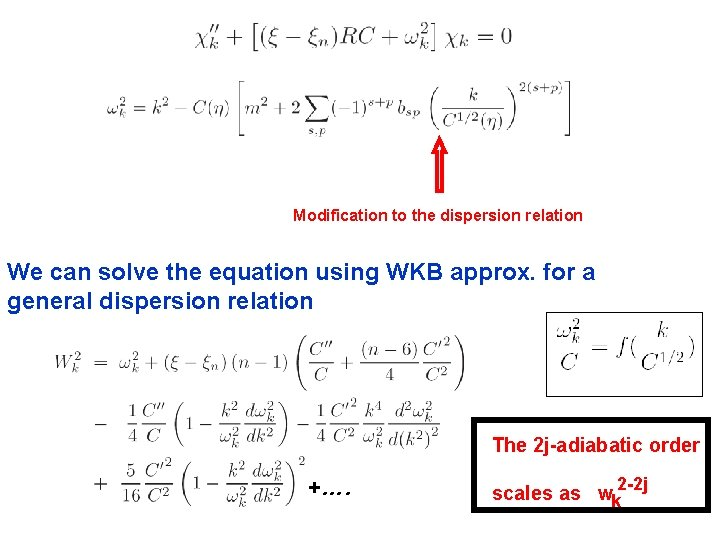Modification to the dispersion relation We can solve the equation using WKB approx. for a general dispersion relation The 2 j-adiabatic order +…. 2 -2 j scales as wk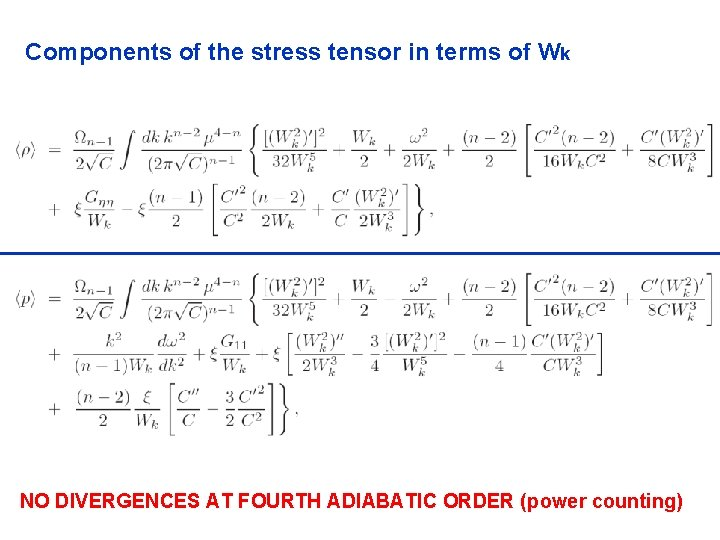Components of the stress tensor in terms of Wk NO DIVERGENCES AT FOURTH ADIABATIC ORDER (power counting)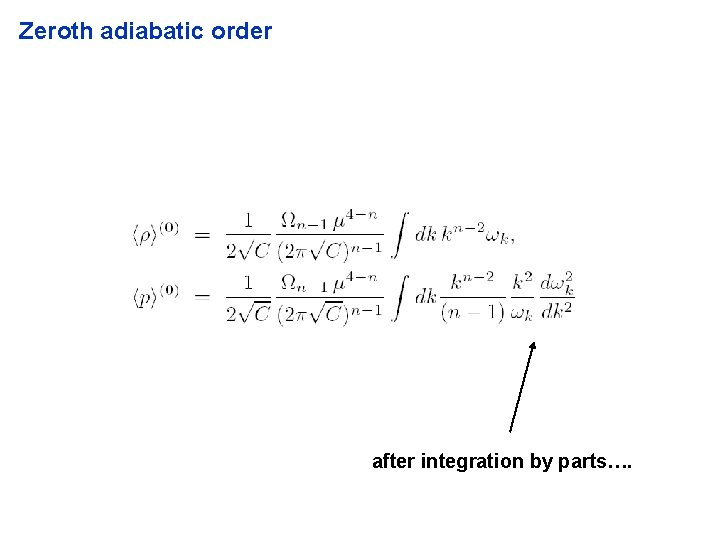Zeroth adiabatic order after integration by parts….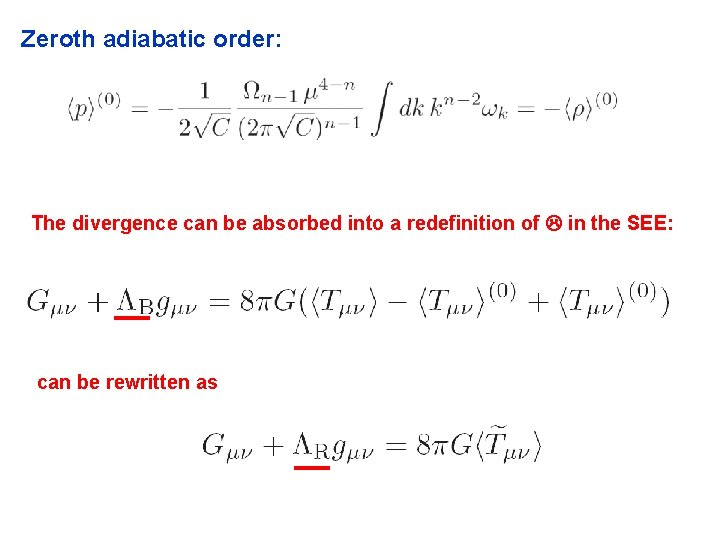Zeroth adiabatic order: The divergence can be absorbed into a redefinition of in the SEE: can be rewritten as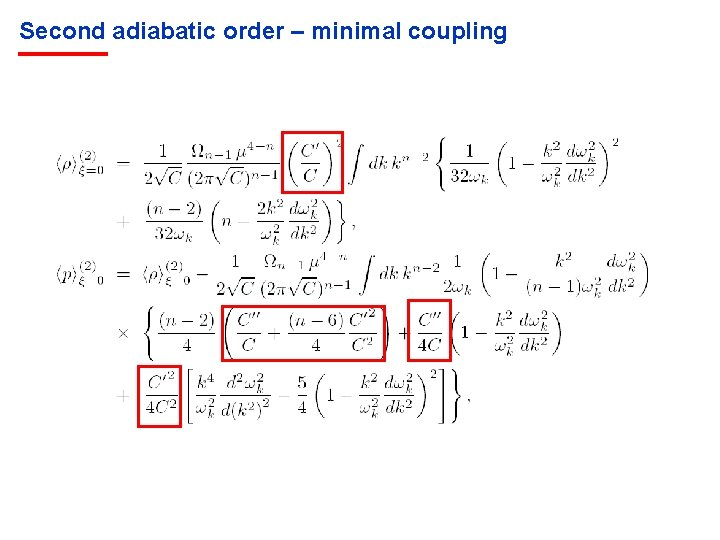Second adiabatic order – minimal coupling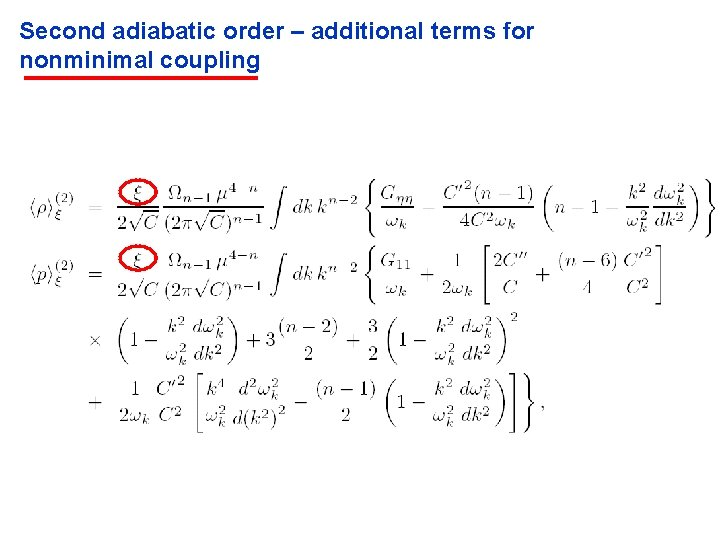Second adiabatic order – additional terms for nonminimal coupling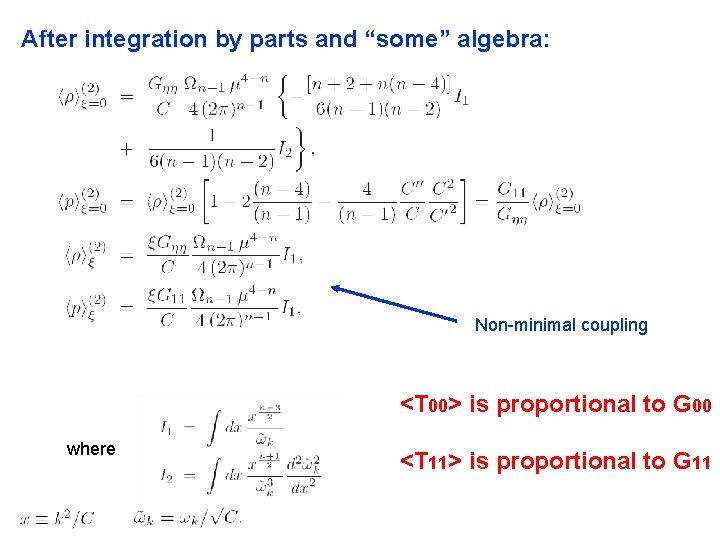After integration by parts and “some” algebra: Non-minimal coupling <T 00> is proportional to G 00 where <T 11> is proportional to G 11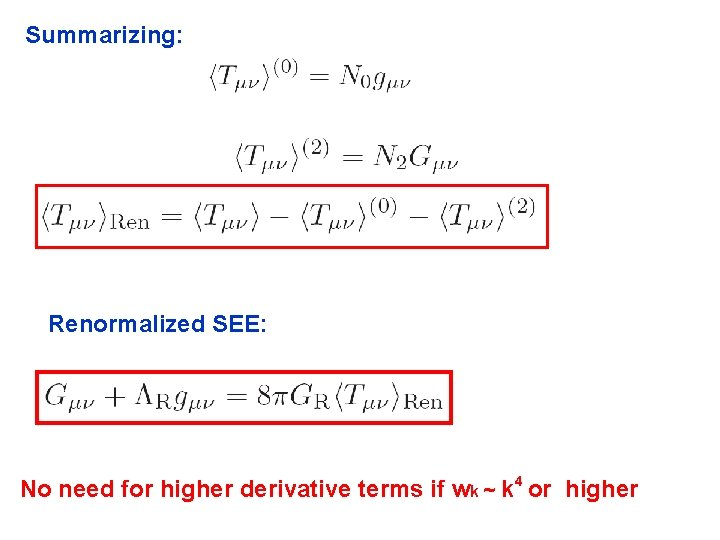Summarizing: Renormalized SEE: No need for higher derivative terms if wk ~ k 4 or higher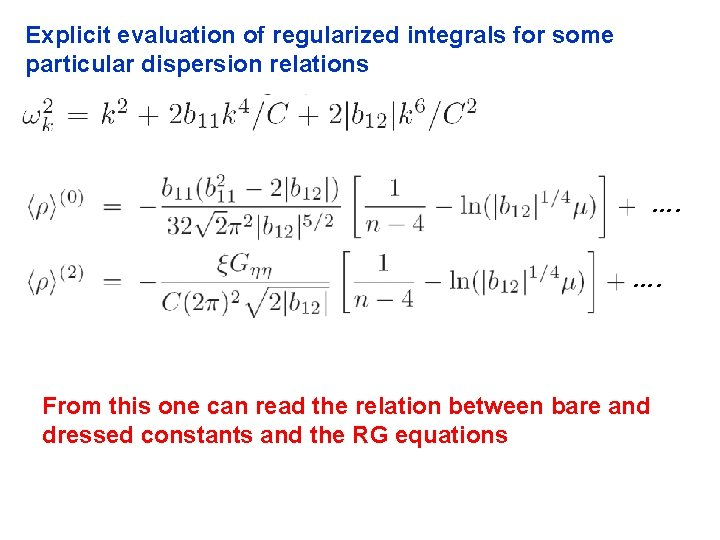Explicit evaluation of regularized integrals for some particular dispersion relations …. …. From this one can read the relation between bare and dressed constants and the RG equationsIn the massless limit If m 0: more complex expressions in terms of Hypergeometric functions Finite results in the limit n->4: similar to usual QFT in 2+1 dimensions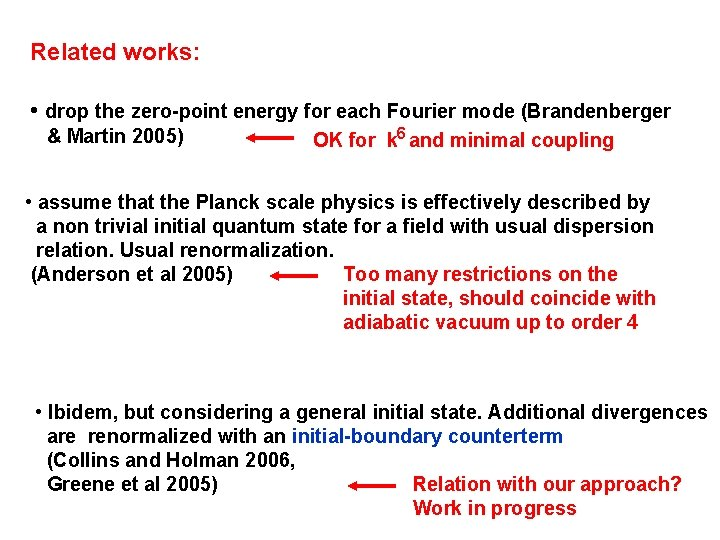Related works: • drop the zero-point energy for each Fourier mode (Brandenberger & Martin 2005) OK for k 6 and minimal coupling • assume that the Planck scale physics is effectively described by a non trivial initial quantum state for a field with usual dispersion relation. Usual renormalization. Too many restrictions on the (Anderson et al 2005) initial state, should coincide with adiabatic vacuum up to order 4 • Ibidem, but considering a general initial state. Additional divergences are renormalized with an initial-boundary counterterm (Collins and Holman 2006, Relation with our approach? Greene et al 2005) Work in progress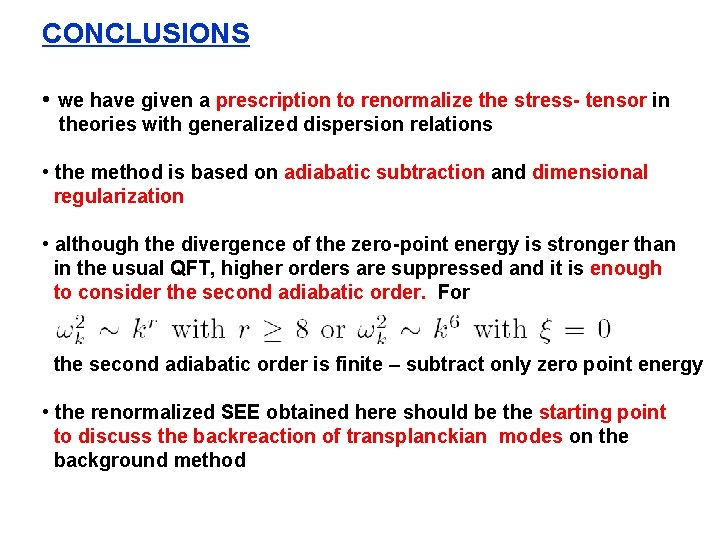CONCLUSIONS • we have given a prescription to renormalize the stress- tensor in theories with generalized dispersion relations • the method is based on adiabatic subtraction and dimensional regularization • although the divergence of the zero-point energy is stronger than in the usual QFT, higher orders are suppressed and it is enough to consider the second adiabatic order. For the second adiabatic order is finite – subtract only zero point energy • the renormalized SEE obtained here should be the starting point to discuss the backreaction of transplanckian modes on the background method